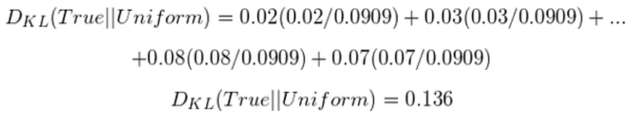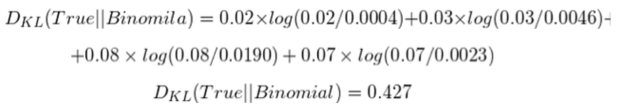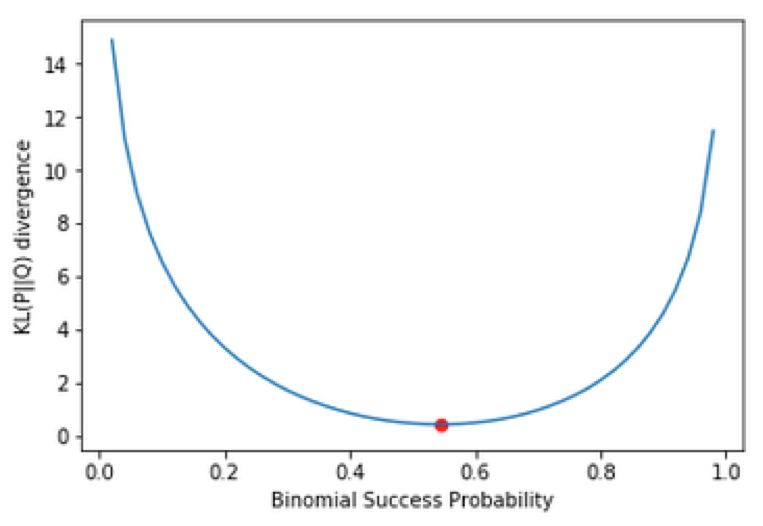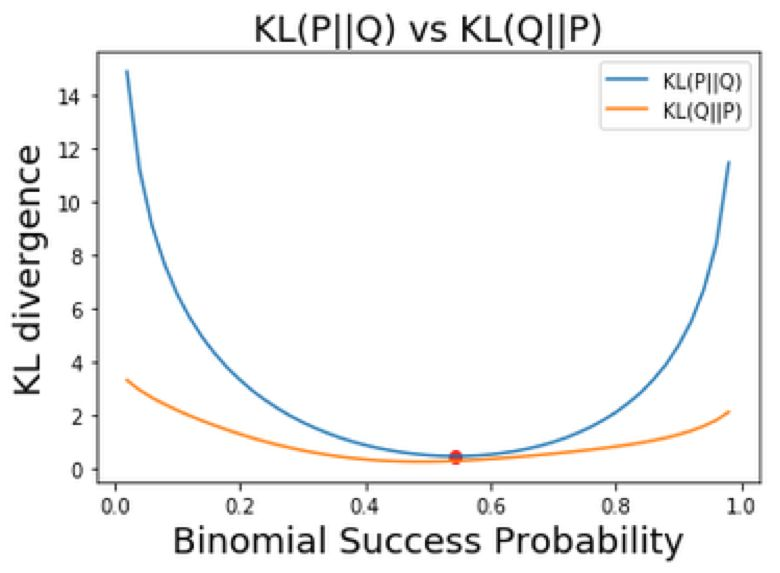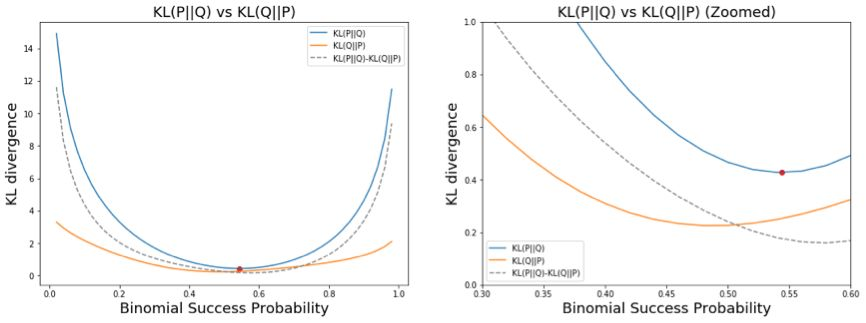# 直观解读KL散度的数学概念

### 基本概念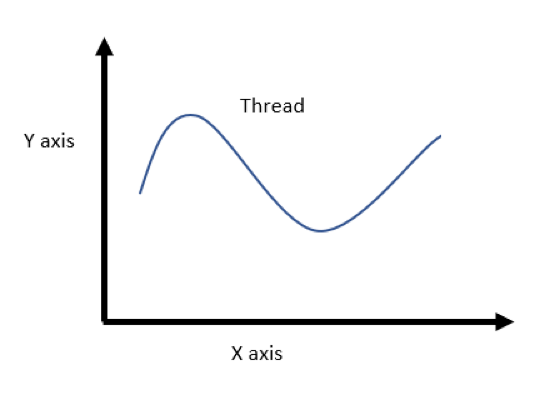### 回到KL散度

0 颗牙齿：2（概率：p_0 = 0.02）

1 颗牙齿：3（概率：p_1 = 0.03）

2 颗牙齿：5（概率：p_2 = 0.05）

3 颗牙齿：14（概率：p_3 = 0.14

4 颗牙齿：16（概率：p_4 = 0.16）

5 颗牙齿：15（概率：p_5 = 0.15）

6 颗牙齿：12（概率：p_6 = 0.12）

7 颗牙齿：8（概率：p_7 = 0.08）

8 颗牙齿：10（概率：p_8 = 0.1）

9 颗牙齿：8（概率：p_9 = 0.08）

10 颗牙齿：7（概率：p_10 = 0.07）

快速做一次完整性检查，确保蠕虫总数为100，且概率总和为1.0.

• 蠕虫总数 = 2+3+5+14+16+15+12+8+10+8+7 = 100

• 概率总和 = 0.02+0.03+0.05+0.14+0.16+0.15+0.12+0.08+0.1+0.08+0.07 = 1.0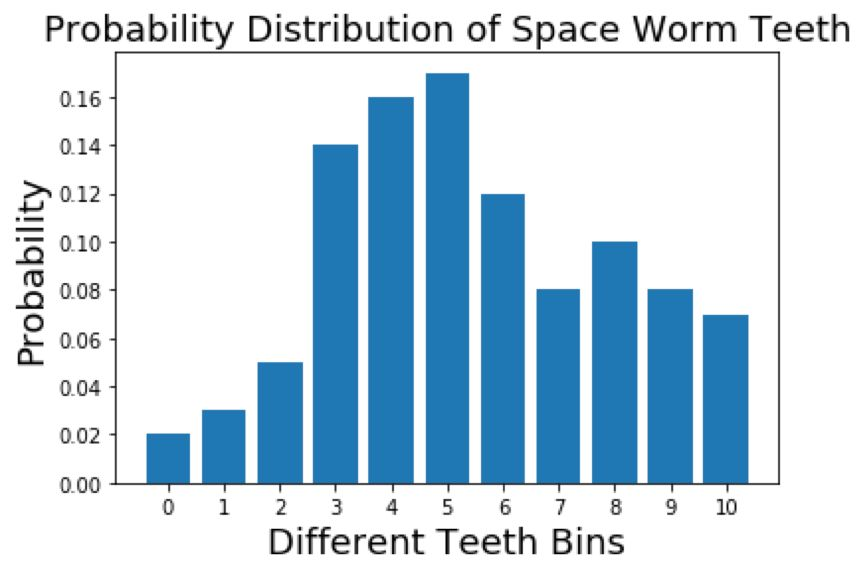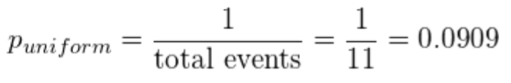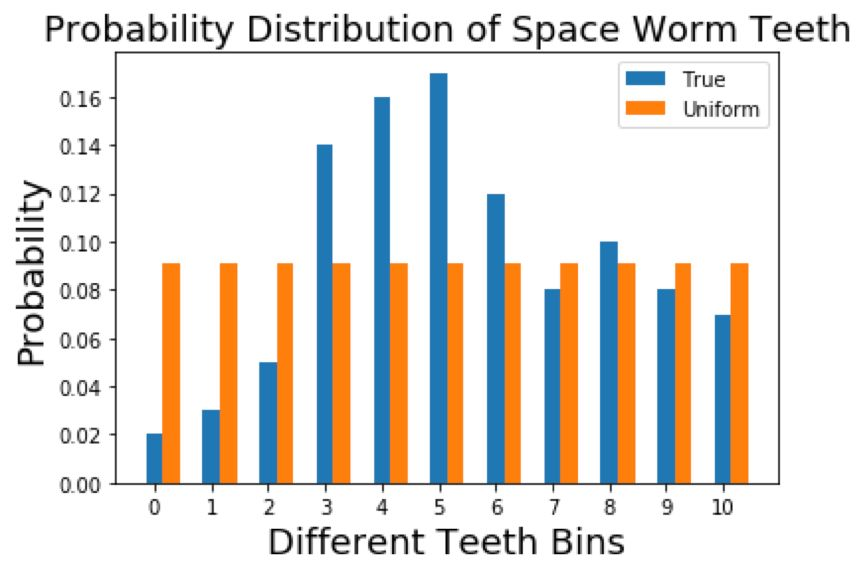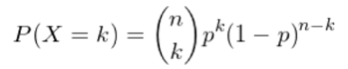0.02*0+1*0.03+2*0.05+3*0.14+4*0.16+5*0.15+6*0.12+7*0.08+8*0.1+9*0.08+10*0.07=5.44

5.44 = 10p

p = 0.544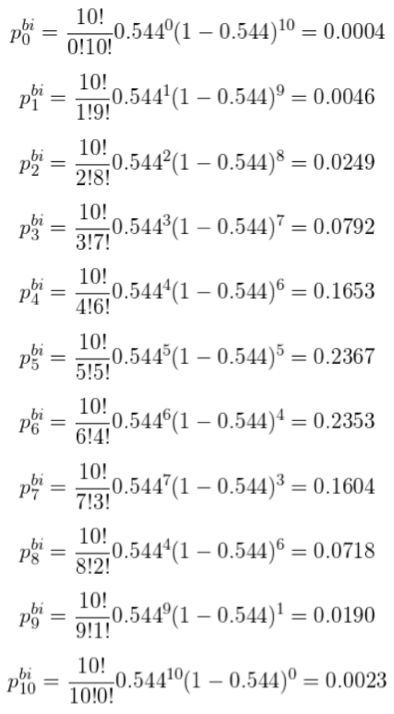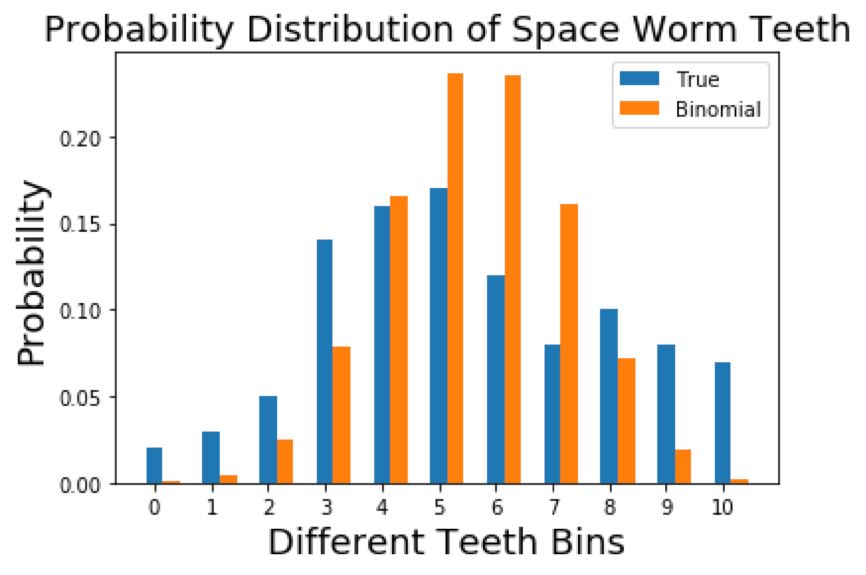• 均匀分布——概率为 0.0909

• 二项分布——n=10、p=0.544，k 取值在 0 到 10 之间。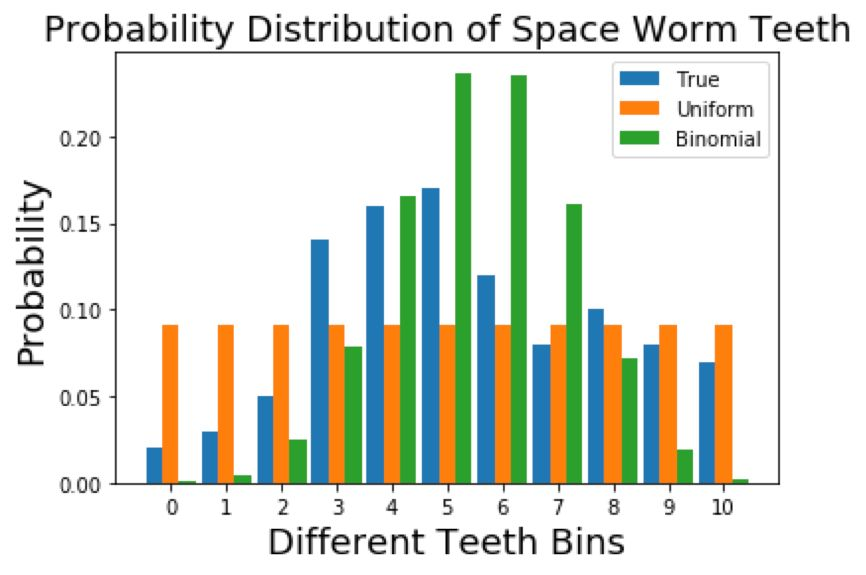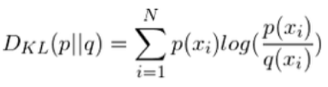KL 散度的直观解释